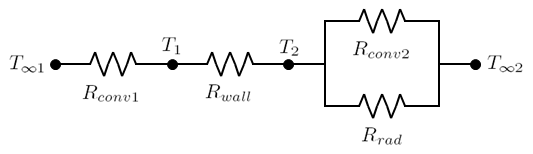f
Neutrium

# Heat Transfer

Heat of Combustion

The heat of combustion is the energy liberated when a substance undergoes complete combustion, at constant pressure usually in an environment with excess Oxygen. The heat of combustion is utilised to quantify the performance of a fuel in a combustion system such as furnaces, power generation turbines and motors. This article describes the heat of combustion and provides a list of heats of combustion for commons fuels and fuel components.

Thermal Conductivity of Metals and Alloys

This article provides thermal conductivity data for a selection of metals and alloys. Thermal conductivity measures a materials ability to allow heat to pass through it via conductance.

Thermal Conductivity of Common Materials

This article provides thermal conductivity data for a selection of common materials. Thermal conductivity measures a materials ability to allow heat to pass through it via conductance.

Thermal ResistanceThe concept of thermal resistance can be utilised to solve steady state heat transfer problems that involve series, parallel or combined series-parallel components. This article demonstrates how to calculate the total thermal resistance for such systems and how to calculate the thermal resistance for practical geometries such as a pipe wall.

Prandtl Number

The Prandtl number is a dimensionless number named after the German physicist Ludwig Prandtl. It represents the ratio of molecular diffusivity of momentum to the molecular diffusivity of heat.

Thermal Contact Resistance

Thermal contact conductance is required to evaluate heat transfer through the interface of two materials and is dependent on a range of material and interface properties. This article provides a brief summary of these properties and a list of thermal contact conductance for a selection of metal-metal pairs.